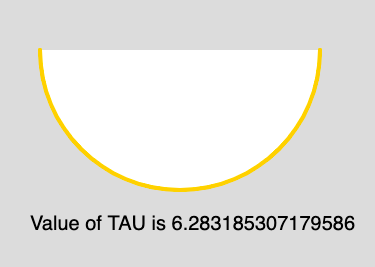# p5.js | Constants | TAU

• Last Updated : 12 Apr, 2019

The TAU is a mathematical constant and it is the alias of TWO_PI. The value of TAU is 6.28318530717958647693. It is twice the ratio of the circumference of a circle to its diameter.

Syntax:

`TAU`

Below program illustrates the usage of TAU in p5.js:

Example: This example illustrates the TAU constant.

 `function` `setup() { ` ` `  `    ``// Create Canvas of size 880*300 ` `    ``createCanvas(880, 300); ` `} ` ` `  `function` `draw() { ` `     `  `    ``//Set the background Color ` `    ``background(220); ` `     `  `    ``//Set the stroke color ` `    ``stroke(255, 204, 0); ` `     `  `    ``//Set the stroke weight ` `    ``strokeWeight(4); ` `     `  `    ``// Use of constant TAU ` `    ``arc(180, 50, 280, 280, 0, TAU/2);  ` `     `  `    ``noStroke(); ` `     `  `    ``// Set the font size ` `    ``textSize(20); ` `     `  `    ``// Display result ` `    ``text(``"Value of TAU is "``+ TAU, 30, 230); ` `} `

Output:Reference: https://p5js.org/reference/#/p5/TAU

My Personal Notes arrow_drop_up
Recommended Articles
Page :